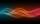# 15 number

What number is smaller (greater) by 15 than its half?

Result

a =  -30
b =  30

#### Solution:

a+15=a/2
b-15=b/2

a = -30
b = 30

a = -30
b = 30

Calculated by our linear equations calculator.

Leave us a comment of example and its solution (i.e. if it is still somewhat unclear...):

Showing 0 comments:Be the first to comment!#### To solve this example are needed these knowledge from mathematics:

Do you have a linear equation or system of equations and looking for its solution? Or do you have quadratic equation?

## Next similar examples:

1. Dropped sheetsThree consecutive sheets dropped from the book. The sum of the numbers on the pages of the dropped sheets is 273. What number has the last page of the dropped sheets?
2. Unknown number 11That number increased by three equals three times itself?
3. Mysterious numberThe magician thinks the number: "The mysterious number is first divided by minus five, dividing the result by three, multiplying the number by ten, and dividing the resulting number by minus four. This gives result 5. Can you reveal the mysterious number?
4. Number with onesThe first digit of the number is 1, if we move this digit to the end we get a 3 times higher number, which is the number?
5. Two numbers 6Fill two natural numbers a, b: 7 + blank- blank = 5
6. Negative in equation2x + 3 + 7x = – 24, what is the value of x?
7. NormThree workers planted 3555 seedlings of tomatoes in one dey. First worked at the standard norm, the second planted 120 seedlings more and the third 135 seedlings more than the first worker. How many seedlings were standard norm?
8. Tickets 3A total of 645 tickets were sold for the school play. They were either adult tickets or student tickets. There were 55 fewer student tickets sold than adult tickets. How many adult tickets were sold?
9. How oldThe student who asked how many years he answered: "After 10 years I will be twice as old than as I was four years ago. How old is student?
10. Forest nurseryIn the forest nursery after winter, they found that 1/10 stems died out of them. For them, they land 193 new spruces. How many spruces are in the forest nursery?
11. Simple equationSolve for x: 3(x + 2) = x - 18
12. Simple equationSolve the following simple equation: 2. (4x + 3) = 2-5. (1-x)
13. Find xSolve: if 2(x-1)=14, then x= (solve an equation with one unknown)
14. EquatiomSolve equation with negatives: X/(-5) + 2 = -9
15. Equation 29Solve next equation: 2 ( 2x + 3 ) = 8 ( 1 - x) -5 ( x -2 )
16. Equation 15Solve equation with variables on both sides:
17. QuizTested student answered correctly on a 4/ 8 questions. Wrong answers was 16. How many questions answered?